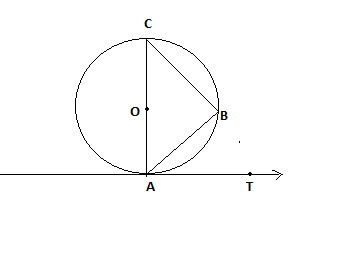QUESTION

# If AB is a chord of a circle with centre O, AOC is a diameter and AT is the tangent at A as shown in figure. Prove that $\angle BAT = \angle ACB$ .Hint: In this question, we use the properties of circle and tangent at any point on the circle. Like we use properties, diameter of a circle subtends a right angle to any point on the circle and tangent to a circle is always perpendicular to the radius.

Given that AC is a diameter and AT is the tangent at A as shown in figure.
Let $\angle BAT = \theta$
Now, we know tangent to a circle is always perpendicular to the radius.
Angle between the tangent AT pass through point A and radius OA is 900.
$\Rightarrow \angle OAT = {90^0} \\ \Rightarrow \angle OAB + \angle BAT = {90^0} \\$
We know, $\angle BAT = \theta$
$\Rightarrow \angle OAB + \theta = {90^0} \\ \Rightarrow \angle OAB = {90^0} - \theta \\$
Now, In $\vartriangle ABC$
$\angle ABC = {90^0}$ (Diameter of a circle subtends a right angle to any point on the circle)
Sum of all angles of $\vartriangle ABC$ is ${180^0}$
$\Rightarrow \angle CAB + \angle ABC + \angle ACB = {180^0}$
As we know, $\angle ABC = {90^0}$ and $\angle OAB = \angle CAB = {90^0} - \theta$
$\Rightarrow {90^0} - \theta + {90^0} + \angle ACB = {180^0} \\ \Rightarrow {180^0} - \theta + \angle ACB = {180^0} \\ \Rightarrow \angle ACB = \theta \\ \Rightarrow \angle ACB = \angle BAT = \theta \\$
Hence proved, $\angle BAT = \angle ACB$

Note: In such types of problems we have to prove LHS (Left hand side) equal to RHS (Right hand side) so we assume LHS angle is $\theta$ and then find the angle of triangle $\vartriangle ABC$ by using the properties of circle and tangent. Then find the RHS angle by using the sum of all angles of triangles equal to ${180^0}$. So, we proved that LHS=RHS.